# Multiplying Negative Fractions

An error occurred trying to load this video.

Try refreshing the page, or contact customer support.

Coming up next: Percentages: Lesson for Kids

### You're on a roll. Keep up the good work!

Replay
Your next lesson will play in 10 seconds
• 0:00 It's All in the Sign
• 0:46 Multiplying Negative Fractions
• 1:30 Let's Practice
• 3:11 Lesson Summary
Save Save

Want to watch this again later?

Timeline
Autoplay
Autoplay
Speed Speed

#### Recommended Lessons and Courses for You

Lesson Transcript
Instructor: Nola Bridgens

Nola has taught elementary school and tutored for four years. She has a bachelor's degree in Elementary Education, a master's degree in Marketing, and is a certified teacher.

It might look intimidating to multiply fractions, but this lesson will break it down into four easy-to-follow steps. We will also discuss important rules to remember when multiplying negatives.

## It's All in the Sign

While multiplying negative fractions may give a negative connotation, it is actually quite simple and can come in handy often. Let's learn how to multiply negative fractions. I'm positive we will have fun.

As long as you pay attention to the sign of the fractions you are multiplying and follow these rules, you will be multiplying negative fractions like a pro in no time!

If both fractions have the same signs, either both positive or both negative, the answer will be positive.

If both fractions have different signs, one positive and one negative, the answer will be negative.

We will explore examples of these rules in the following sections.

## Multiplying Negative Fractions

Here are the four steps to multiply negative fractions. Let's use the following problem to illustrate the steps.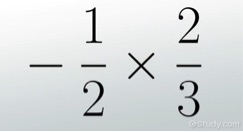Remember that the numerators are the numbers over the bar in a fraction and the denominators are the numbers under the bar in fractions.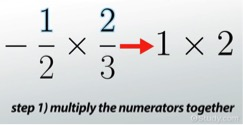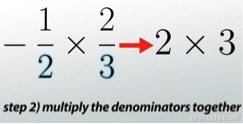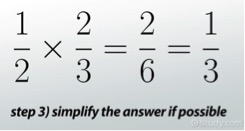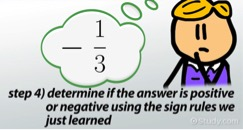Remember, a whole number can be turned into a fraction by simply placing the whole number over 1. For example, the whole number 7 becomes 7/1.

## Let's Practice

Let's give it a try together. Let's find the product of -2/7 * 3/4

Step 1) When we multiply the numerators (2 * 3) the answer is 6.

Step 2) When we multiply the denominators (7 * 4) the answer is 28.

Step 3) The new fraction is 6/28. Both numbers are divisible by 2 and can be simplified to 3/14.

To unlock this lesson you must be a Study.com Member.

### Register to view this lesson

Are you a student or a teacher?

#### See for yourself why 30 million people use Study.com

##### Become a Study.com member and start learning now.
Back
What teachers are saying about Study.com

### Earning College Credit

Did you know… We have over 200 college courses that prepare you to earn credit by exam that is accepted by over 1,500 colleges and universities. You can test out of the first two years of college and save thousands off your degree. Anyone can earn credit-by-exam regardless of age or education level.